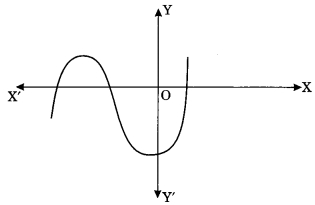Check the below NCERT MCQ Questions for Class 10 Maths Chapter 2 Polynomials with Answers Pdf free download. MCQ Questions for Class 10 Maths with Answers were prepared based on the latest exam pattern. We have provided Polynomials Class 10 Maths MCQs Questions with Answers to help students understand the concept very well. https://ncertmcq.com/mcq-questions-for-class-10-maths-with-answers/

Students can also refer to NCERT Solutions for Class 10 Maths Chapter 2 Polynomials for better exam preparation and score more marks.

## Polynomials Class 10 MCQs Questions with Answers

Polynomials Class 10 MCQ Question 1.
The maximum number of zeroes that a polynomial of degree 4 can have is
(a) One
(b) Two
(c) Three
(d) Four

Polynomial root calculator imaginary numbers. Welcome to our step-by-step math solver! Solve ·

Polynomial Class 10 MCQ Question 2.
The graph of the polynomial p(x) = 3x – 2 is a straight line which intersects the x-axis at exactly one point namely
(a) ($$\frac{-2}{3}$$, 0)
(b) (0, $$\frac{-2}{3}$$)
(c) ($$\frac{2}{3}$$, 0)
(d) $$\frac{2}{3}$$, $$\frac{-2}{3}$$

Answer: (c) ($$\frac{2}{3}$$, 0)

polynomial calculator that will find solution, step-by-step online.

Class 10 Maths Chapter 2 MCQ Question 3.
In fig. given below, the number of zeroes of the polynomial f(x) is(a) 1
(b) 2
(c) 3
(d) None

Class 10 Polynomials MCQ Question 4.
The graph of the polynomial ax² + bx + c is an upward parabola if
(a) a > 0
(b) a < 0
(b) a = 0
(d) None

Polynomial MCQ Class 10 Question 5.
The graph of the polynomial ax² + bx + c is a downward parabola if
(a) a > 0
(b) a < 0
(c) a = 0
(d) a = 1

MCQ On Polynomials Class 10 Question 6.
A polynomial of degree 3 is called
(a) a linear polynomial
(c) a cubic polynomial

Polynomials MCQ Class 10 Question 7.
If α, β are the zeroes of the polynomial x² – 16, then αβ(α + β) is
(a) 0
(b) 4
(c) -4
(d) 16

MCQ Of Polynomials Class 10 Question 8.
If α and $$\frac{1}{α}$$ are the zeroes of the polynomial ax² + bx + c, then value of c is
(a) 0
(b) a
(c) -a
(d) 1

Polynomials Class 10 MCQ With Answers Question 9.
Zeroes of the polynomial x² – 11 are
(a) ±$$\sqrt{17}$$
(b) ±$$\sqrt{3}$$
(c) 0
(d) None

Answer: (a) ±$$\sqrt{17}$$

Ch 2 Maths Class 10 MCQ Question 10.
If α, β, γ are the zeroes of the cubic polynomial ax³ + bx² + cx + d then α + β + γ is equal
(a) $$\frac{-b}{a}$$
(b) $$\frac{b}{a}$$
(c) $$\frac{c}{a}$$
(d) $$\frac{d}{a}$$

Answer: (a) $$\frac{-b}{a}$$

MCQ Questions For Class 10 Maths Chapter 2 Question 11.
If α, β, γ are the zeroes of the cubic polynomial ax³ + bx² + cx + d then αβ + βγ + αγ is equal to
(a) $$\frac{-b}{a}$$
(b) $$\frac{b}{a}$$
(c) $$\frac{c}{a}$$
(d) $$\frac{d}{a}$$

Answer: (c) $$\frac{c}{a}$$

Chapter 2 Maths Class 10 MCQ Question 12.
If the zeroes of the polynomial x³ – 3x² + x – 1 are $$\frac{s}{t}$$, s and st then value of s is
(a) 1
(b) -1
(c) 2
(d) -3

Class 10 Maths Ch 2 MCQ Question 13.
If the sum of the zeroes of the polynomial f(x) = 2x³ – 3kx² + 4x – 5 is 6, then the value of k is
(a) 2
(b) 4
(c) -2
(d) -4

Class 10 Maths Polynomials MCQ Question 14.
If a polynomial of degree 4 is divided by quadratic polynomial, the degree of the remainder is
(a) ≤ 1
(b) ≥ 1
(c) 2
(d) 4

Class 10 Maths Chapter 2 MCQ With Answers Question 15.
If a – b, a and a + b are zeroes of the polynomial fix) = 2x³ – 6x² + 5x – 7, then value of a is
(a) 1
(b) 2
(c) -5
(d) 7

Question 16.
Dividend is equal to
(a) divisor × quotient + remainder
(b) divisior × quotient
(c) divisior × quotient – remainder
(d) divisor × quotient × remainder

Answer: (a) divisor × quotient + remainder

Question 17.
A quadratic polynomial whose sum of the zeroes is 2 and product is 1 is given by
(a) x² – 2x + 1
(b) x² + 2x + 1
(c) x² + 2x – 1
(d) x² – 2x – 1

Answer: (a) x² – 2x + 1

Question 18.
If one of the zeroes of a quadratic polynomial ax² + bx + c is 0, then the other zero is
(a) $$\frac{-b}{a}$$
(b) 0
(c) $$\frac{b}{a}$$
(d) $$\frac{-c}{a}$$

Answer: (a) $$\frac{-b}{a}$$

Question 19.
The sum and the product of the zeroes of polynomial 6x² – 5 respectively are
(a) 0, $$\frac{-6}{5}$$
(b) 0, $$\frac{6}{5}$$
(c) 0, $$\frac{5}{6}$$
(d) 0, $$\frac{-5}{6}$$

Answer: (d) 0, $$\frac{-5}{6}$$

Question 20.
What should be subtracted from x³ – 2x² + 4x + 1 to get 1?
(a) x³ – 2x² + 4x
(b) x³ – 2x² + 4 + 1
(c) -1
(d) 1